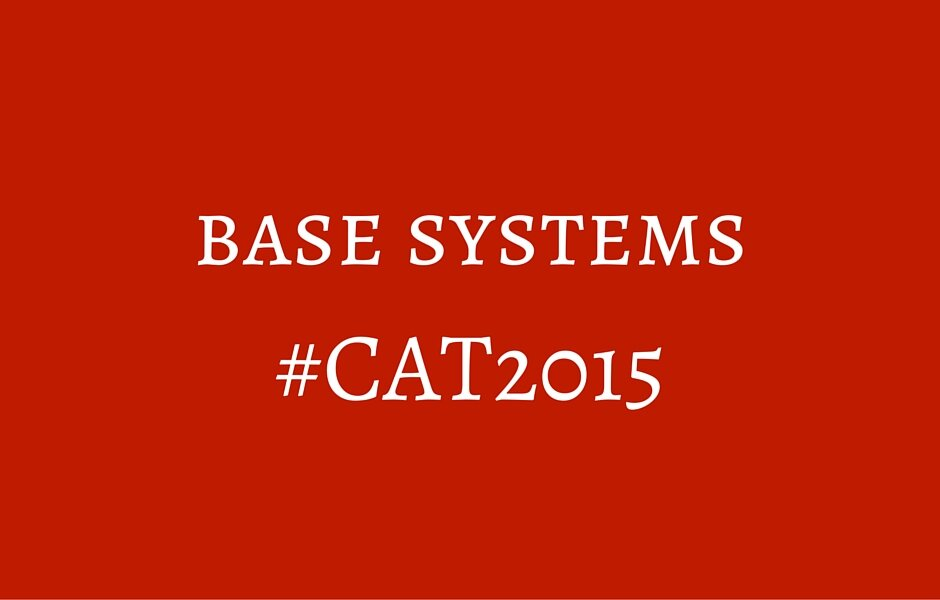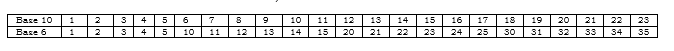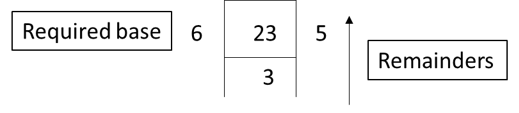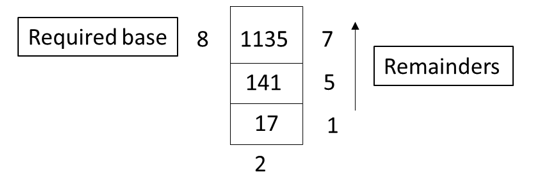Select PageThe concept is probably the easiest to understand as it deals with the basics of counting but is found to be confusing by many aspirants. Let us take a look at the concepts of conversion of bases and also a few question types that appeared in previous year CAT papers and other entrance tests.

What are base systems?

It is probably a commonplace notion that the system of counting began with our ancestors who used 10 digits (literally) to represent different values. With the need to have a formal system for counting, the decimal number system became the easiest and the most widespread system.

Questions involving base systems, in their most basic format, try to figure out what would be our lives like if we had a different number of digits. The basics would remain the same; just that we would have adapted to that system of counting. So, for example, if instead of 10 digits, we had 9 digits, our counting system would have looked something like:

0, 1, 2, 3, 4, 5, 6, 7, 8, 10, 11, 12, 13, 14, 15, 16, 17, 18, 20 and so on.

It is pretty easy to visualize that the digit 9 is not present and so, as soon as we reach 8, we have exhausted all the single digits and so, would need to make the smallest two-digit number. In this scenario, the highest single digit number is 8, the highest two digit number is 88, the highest three digit number is 888 and so on.

What exactly happened in this case? It is a matter of simple combinations. If you have one digit to write and you have 10 options, you can write that digit in 10 ways. If you have to write a two digit number using 10 different digits, one of which is 0, it can be done by visualizing that the first digit cannot be 0 and so, can be chosen in 9 ways and the second digit can be anything from 0 to 9 and so, can be chosen in 10 ways. So, a total of 90 two digit numbers can be formed.

Now, if a number 243 is written in the decimal system, we expand it as

2*100 + 4*10 + 3*1

Now, this is nothing but 2*102 + 4*101 + 3*100

This is 10 because there are 10 digits. If there were 9 digits and the number had to be represented, it would be 2*92 + 4*91 + 3*90

Conversion from decimal to other base systems

Most of the questions in CAT involve converting numbers from one base system to other. If a number is in the decimal system and has to be converted to another base, it simply means that you count the number in the new base. For example: if there is a number 23 that has to be converted to base 6, it would look as follows:As you can see, for every 10 numbers in the first row, you have 6 numbers in the second row. The method involves using this and finding successive remainders on division by 6.We simply divide the number by 6 and keep on doing it till the time it is no longer divisible. Then we start from the bottom and move to the top writing down all the remainders in that order.

Let’s try a larger number. What is the base 8 equivalent of 1135 in decimal notation?So, the same number in base 8 will be 2157. With practice, you can skip a couple of steps and simply start writing it as shown in the images.

Conversion from other base systems to decimal

This is simpler and many a time, questions come from this area wherein, operations will be performed on numbers in particular bases and then, the parent base will have to be identified. Conversion is a part of the exercise and is pretty easy. Let’s see this with the help of an example.

A number in base 6 is 3254. What is the decimal equivalent of the number?

As we saw, in base n notation, the positions will be multiplied by n0, n1, n2, and so on. So, if a number is present in base 6, the base 10 equivalent will be got simply by multiplying the number in each position with the corresponding base.

So, (3254)6 = 3*63 + 2*62 + 5*61 + 4*60 = 648+72+30+4 = 754.

PS: Remember that a base 6 number cannot have the digit 6 in it, similarly, a base 7 cannot have the digit 7 in it and so on. In cases where you would get multiple cases, you have to rule out these in case they are one of your solutions.

Honorary mention – Josephus’ puzzle

While it does not really appear in CAT and was asked once in XAT 2011 (probably the toughest paper in the history of MBA entrances) it would serve you to know the concept.

The question would be something like: 100 people are standing in a circle at positions 1 to 100. A sword is given to the person on the first position who kills the second person and hands the sword to the third person who kills the fourth person and so on. What would be the position of the survivor?

The original story is very interesting but the solution remains simple. You simply convert the total number of people to base 2, then shift the leftmost digit to the rightmost position and then convert it back to decimal and voila! In this case, 100 will be represented in binary as 1100100. Shifting the leftmost digit (obviously 1) to the rightmost position will give us 1001001 which in decimal notation is 73 (the best number!). So, if you find yourself in such a predicament, 73 is the place to be.

Examples from previous year CAT papers:

Convert the number 1982 from base 10 to base 12. The result is (CAT 2000)

(a) 1182      (b) 1912      (c) 1192      (d) 1292

In a number system the product of 44 and 11 is 3414. The number 3111 of this system, when converted to the decimal number system, becomes (CAT 2001)

(a) 406        (b) 1086      (c) 213        (d) 691

Let the base system be n. So, (3414)n = (484)10

3n3 + 4n2 + n + 4 = 484

3n3 + 4n2 + n = 480

By simple observation, you can figure out that 5 satisfies the equation and so, the number system should be in base 5. So, (3111)5 = 375+25+5+1 = 406.

A positive whole number M less than 100 is represented in base 2 notation, base 3 notation, and base 5 notation. It is found that in all three cases the last digit is 1, while in exactly two out of the three cases the leading digit is 1. Then M equals (CAT 2003 Leaked)

(a) 31           (b) 63           (c) 75           (d) 91

How many 7 digit numbers are there in base 2? How many of these are divisible by 8 in base 10?

A slightly difficult question which requires basics of numbers and P&C to solve it. Try it once and drop us a comment with the solution/approach.

You can go through all the other articles in the 75 days to CAT series as well.

All the best!

error: Content is protected !!